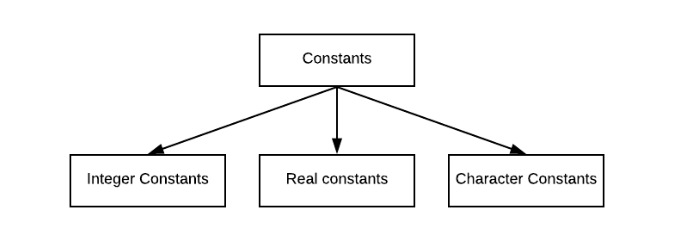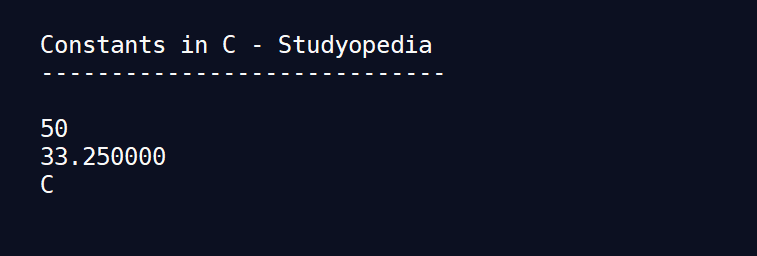# Constants in C Language

## 27 Aug Constants in C Language

Constants in C language are the values that cannot be modified once they are defined. After definition, they are fixed in a program. There are primarily three types of constants, namely integer, real and character constants.Details about these are given as follows:

## Integer Constants

The different rules for constructing integer constants are:

1. There should be at least one digit in the integer constant.
2. There should be no decimal points.
3. The integer constant can be positive or negative.
4. If there is no sign specified before the integer constant, it is assumed to be positive.
5. There should be no commas and blanks in the integer constant.

Some examples of integer constants are 23,-55,+55 etc.

## Real Constants

Real constants or floating point constants can be written in two forms: Fractional form or Exponential form.

The different rules for constructing real constants in fractional form are:

1. There should be at least one digit in the real constant.
2. There should be a decimal point.
3. The real constant can be positive or negative.
4. If there is no sign specified before the real constant, it is assumed to be positive.
5. There should be no commas and blanks in the real constant.

Some examples of real constants in fractional form are 23.5, +66.87, -75.23 etc.

The exponential form is usually used when the real constants are either too large or too small. In this form, the constants are represented in two parts. The part before e is called mantissa and the part after e is called exponent.

The different rules for constructing real constants in exponential form are:

1. e should separate the mantissa and exponent.
2. The mantissa can be positive or negative.
3. If there is no sign specified before the mantissa, it is assumed to be positive.
4. There should be at least one digit in the exponent. It is assumed to be positive by default.

Some examples of real constants in exponential form are +2.3e5, -6.6e-7, -7.5e3 etc.

## Character Constants

These consist of a single character such as an alphabet, digit or special character enclosed within inverted commas.

The different rules for constructing character constants are:

1. Maximum length of a character constant is one character.
2. The character constant should be enclosed with left pointing inverted commas.
3. Any alphabet, digit or special character can be a character constant.

Some examples of character constants in are ‘A’, ‘7’, ‘%’  etc.

A coding example of constants in C language is:

The output obtained for the above code is:[email protected]

We work to create free tutorials for all.